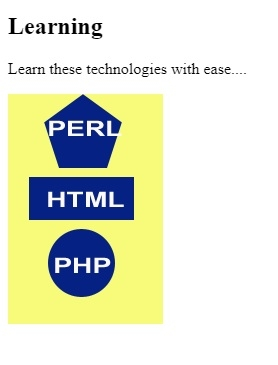# HTML <area> alt Attribute

HTMLWeb DevelopmentFront End Technology

The alt attribute of <area> element is used to set an alternate text for an area. This alternate text i.e. alt is visible when the image isn’t displayed for reasons like internet, loading issues, errors, etc.

Following is the syntax −

<area alt="text">

Here, text is the alt text to be set.

Let us now see an example to implement the alt attribute of the <area> element −

## Example

Live Demo

<!DOCTYPE html>
<html>
<body>
<h2>Learning</h2>
<p>Learn these technologies with ease....</p>
<img src = /images/usemap.gif alt = "usemap" border = "0" usemap = "#tutorials"/>
<map name = "tutorials">
<area shape = "poly" coords = "74,0,113,29,98,72,52,72,38,27"
href = "/perl/index.htm" alt = "Perl Tutorial" target = "_blank" />
<area shape = "rect" coords = "22,83,126,125" alt = "HTML Tutorial"
href = "/html/index.htm" target = "_blank" />
<area shape = "circle" coords = "73,168,32" alt = "PHP Tutorial"
href = "/php/index.htm" target = "_blank" />
</map>
</body>
</html>

## OutputIn the above example, we have set the map on the following image −

<img src = /images/usemap.gif alt = "usemap" border = "0" usemap = "#tutorials"/>

Now, we have set the map and area within it for shape −

<map name = "tutorials">
<area shape = "poly" coords = "74,0,113,29,98,72,52,72,38,27"
href = "/perl/index.htm" alt = "Perl Tutorial" target = "_blank" />
<area shape = "rect" coords = "22,83,126,125" alt = "HTML Tutorial"
href = "/html/index.htm" target = "_blank" />
<area shape = "circle" coords = "73,168,32" alt = "PHP Tutorial"
href = "/php/index.htm" target = "_blank" />
</map>

While setting the area, the alt is also set using alt attribute −

<area shape = "poly" coords = "74,0,113,29,98,72,52,72,38,27" href = "/perl/index.htm" alt = "Perl Tutorial" target = "_blank" />